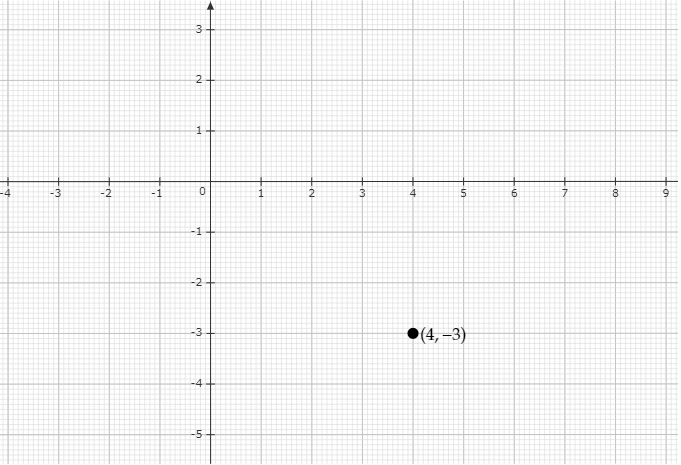# Plot the following points on the graph paper:$(4, -3)$

Given:

$(4, -3)$

To do:

We have to plot the given point on the graph paper.

Solution:

The given point can be plotted on the graph paper as below: